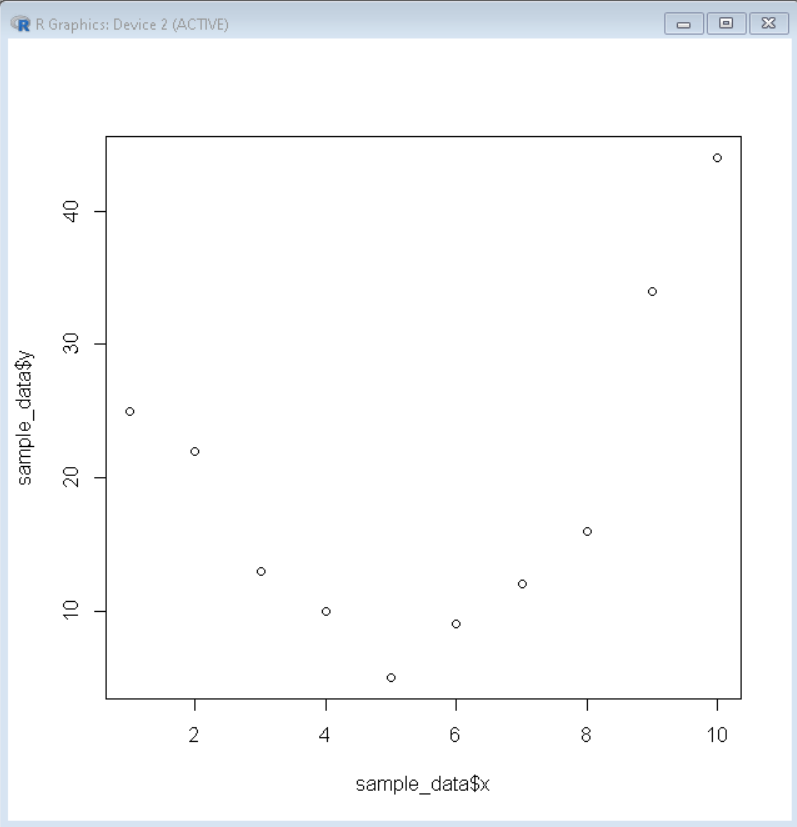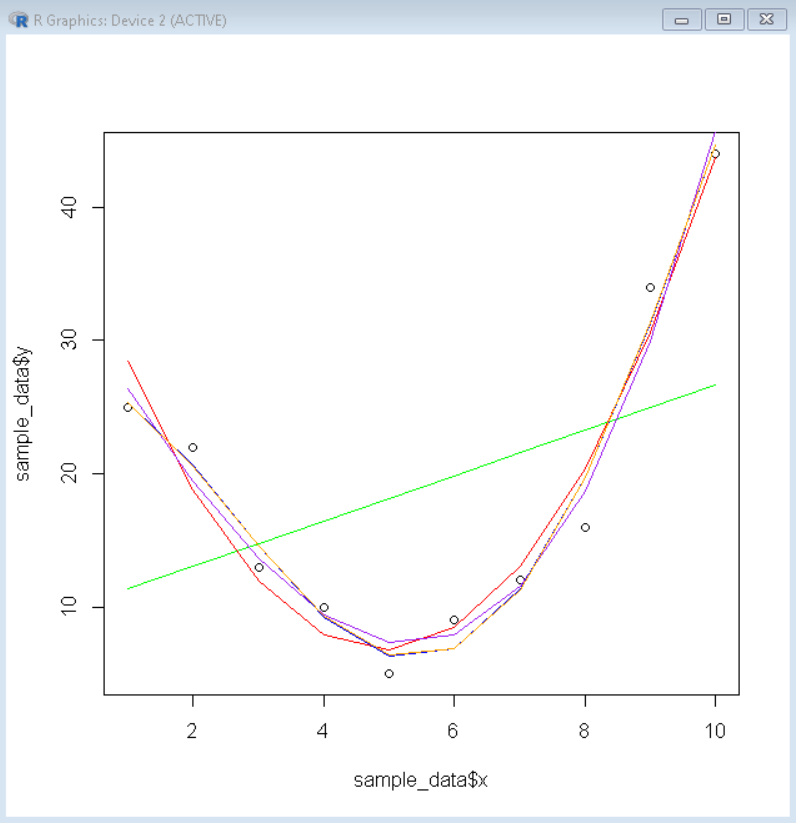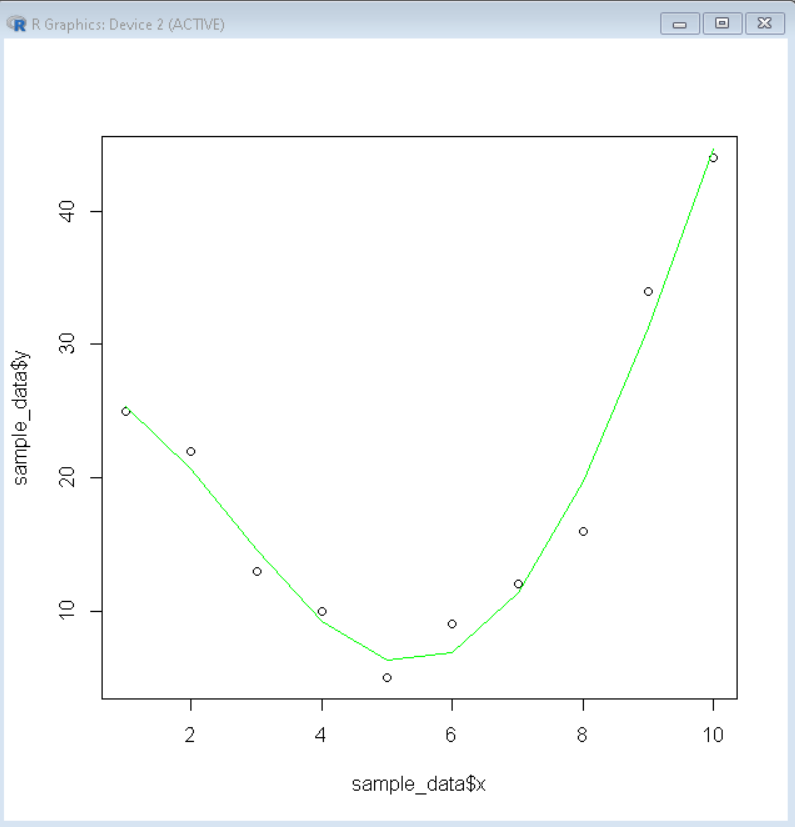# Curve Fitting in R

• Last Updated : 19 Dec, 2021

In this article, we will discuss how to fit a curve to a dataframe in the R Programming language.

Curve fitting is one of the basic functions of statistical analysis. It helps us in determining the trends and data and helps us in the prediction of unknown data based on a regression model/function.

## Visualization of Dataframe:

To fit a curve to some data frame in the R Language we first visualize the data with the help of a basic scatter plot. In the R language, we can create a basic scatter plot by using the plot() function.

Syntax:

`plot( df\$x, df\$y)`

where,

• df: determines the data frame to be used.
• x and y: determines the axis variables.

Example:

## R

 `# create sample data ` `sample_data <- ``data.frame``(x=1:10, ` `                 ``y=``c``(25, 22, 13, 10, 5,  ` `                     ``9, 12, 16, 34, 44)) ` ` `  `#create a basic scatterplot  ` `plot``(sample_data\$x, sample_data\$y)`

Output:## Create Several Curves to fit on data

Then we create linear regression models to the required degree and plot them on top of the scatter plot to see which one fits the data better. We use the lm() function to create a linear model. And then use lines() function to plot a line plot on top of scatter plot using these linear models.

Syntax:

`lm(  function, data)`

where,

• function: determines the fitting polynomial function.
• data: determines the data frame over which function is to be fitted.

Example:

## R

 `# create sample data ` `sample_data <- ``data.frame``(x=1:10, ` `                 ``y=``c``(25, 22, 13, 10, 5,  ` `                     ``9, 12, 16, 34, 44)) ` ` `  `# fit polynomial regression models up to degree 5 ` `linear_model1 <- ``lm``(y~x, data=sample_data) ` `linear_model2 <- ``lm``(y~``poly``(x,2,raw=``TRUE``), data=sample_data) ` `linear_model3 <- ``lm``(y~``poly``(x,3,raw=``TRUE``), data=sample_data) ` `linear_model4 <- ``lm``(y~``poly``(x,4,raw=``TRUE``), data=sample_data) ` `linear_model5 <- ``lm``(y~``poly``(x,5,raw=``TRUE``), data=sample_data) ` ` `  `# create a basic scatterplot  ` `plot``(sample_data\$x, sample_data\$y) ` ` `  `# define x-axis values ` `x_axis <- ``seq``(1, 10, length=10) ` ` `  `# add curve of each model to plot ` `lines``(x_axis, ``predict``(linear_model1, ``data.frame``(x=x_axis)), col=``'green'``) ` `lines``(x_axis, ``predict``(linear_model2, ``data.frame``(x=x_axis)), col=``'red'``) ` `lines``(x_axis, ``predict``(linear_model3, ``data.frame``(x=x_axis)), col=``'purple'``) ` `lines``(x_axis, ``predict``(linear_model4, ``data.frame``(x=x_axis)), col=``'blue'``) ` `lines``(x_axis, ``predict``(linear_model5, ``data.frame``(x=x_axis)), col=``'orange'``)`

Output:## Best fit curve with adjusted r squared value

Now since we cannot determine the better fitting model just by its visual representation, we have a summary variable r.squared this helps us in determining the best fitting model. The adjusted r squared is the percent of the variance of Y intact after subtracting the error of the model. The more the R Squared value the better the model is for that data frame. To get the adjusted r squared value of the linear model, we use the summary() function which contains the adjusted r square value as variable adj.r.squared.

Syntax:

`summary( linear_model )\$adj.r.squared`

where,

• linear_model: determines the linear model whose summary is to be extracted.

Example:

## R

 `# create sample data ` `sample_data <- ``data.frame``(x=1:10, ` `                 ``y=``c``(25, 22, 13, 10, 5,  ` `                     ``9, 12, 16, 34, 44)) ` ` `  `# fit polynomial regression models up to degree 5 ` `linear_model1 <- ``lm``(y~x, data=sample_data) ` `linear_model2 <- ``lm``(y~``poly``(x,2,raw=``TRUE``), data=sample_data) ` `linear_model3 <- ``lm``(y~``poly``(x,3,raw=``TRUE``), data=sample_data) ` `linear_model4 <- ``lm``(y~``poly``(x,4,raw=``TRUE``), data=sample_data) ` `linear_model5 <- ``lm``(y~``poly``(x,5,raw=``TRUE``), data=sample_data) ` ` `  `# calculated adjusted R-squared of each model ` `summary``(linear_model1)\$adj.r.squared ` `summary``(linear_model2)\$adj.r.squared ` `summary``(linear_model3)\$adj.r.squared ` `summary``(linear_model4)\$adj.r.squared ` `summary``(linear_model5)\$adj.r.squared `

Output:

``` 0.07066085
 0.9406243
 0.9527703
 0.955868
 0.9448878```

## Visualize Best fit curve with data frame:

Now since from the above summary, we know the linear model of fourth-degree fits the curve best with an adjusted r squared value of 0.955868. So, we will visualize the fourth-degree linear model with the scatter plot and that is the best fitting curve for the data frame.

Example:

## R

 `# create sample data ` `sample_data <- ``data.frame``(x=1:10, ` `                          ``y=``c``(25, 22, 13, 10, 5, ` `                              ``9, 12, 16, 34, 44)) ` ` `  `# Create best linear model ` `best_model <- ``lm``(y~``poly``(x,4,raw=``TRUE``), data=sample_data) ` ` `  `# create a basic scatterplot  ` `plot``(sample_data\$x, sample_data\$y) ` ` `  `# define x-axis values ` `x_axis <- ``seq``(1, 10, length=10) ` ` `  `# plot best model ` `lines``(x_axis, ``predict``(best_model, ``data.frame``(x=x_axis)), col=``'green'``)`

Output:My Personal Notes arrow_drop_up
Recommended Articles
Page :